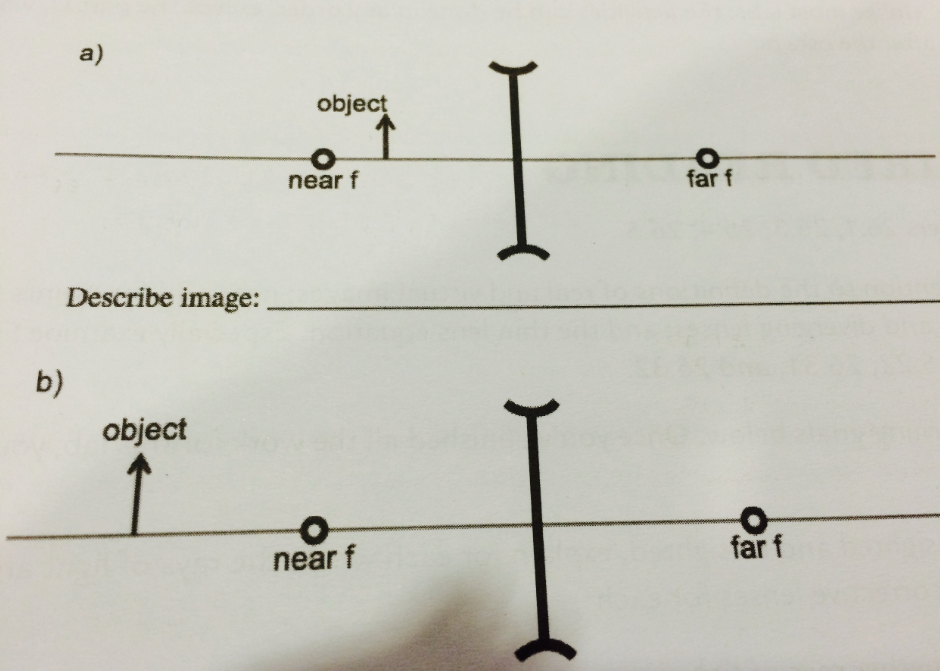# Problem: Two situations involving objects and diverging lenses are given. Draw the three principle rays for these two situations. Also draw the image on the ray diagram where the three principal rays converging and then describe the image (i.e. relative location, magnification, orientation).

###### FREE Expert Solution

The three principal rays are:

i. A ray from the top of the object parallel to the principal axis perpendicular to the lens.

ii. A ray through the center of the lens.

iii. A ray through the principal focal point on the near side of the lens.

These rays are shown in the figures:

97% (380 ratings)###### Problem Details

Two situations involving objects and diverging lenses are given. Draw the three principle rays for these two situations. Also draw the image on the ray diagram where the three principal rays converging and then describe the image (i.e. relative location, magnification, orientation).